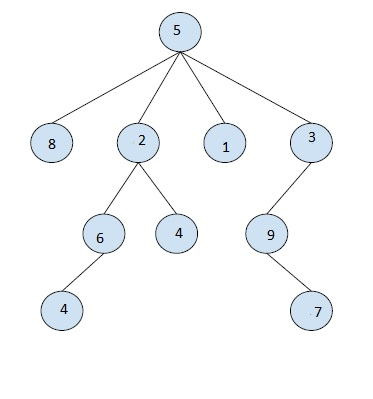# Find the Kth node in the DFS traversal of a given subtree in a Tree in C++

In this problem, we are given a tree of size N, a node of the tree V and k. Our task is find the Kth node in the DFS traversal of a given subtree in a Tree.

We need to find the kth node in the DFS traversal of the tree starting from vertex V.

Let's take an example to understand the problem,

Input :V = 2, k = 3

Output : 4

Explanation

The series is {1, 2, 3, 5, 6, 7}
The 4th element is 5.

## Solution Approach

A simple solution to the problem is find the DFS traversal of the node V and print the kth value from this.

For this, we perform DFS traversal of the tree and store it in a vector. In this vector, we will find values for Vstart and Vend marking the start and end of the DFS traversal of the tree. Then find the k-th value in this range and print it if possible.

## Example

Program to illustrate the working of our solution

#include <bits/stdc++.h>
using namespace std;
#define N 100005
int n;
vector<int> tree[N];
int currentIdx;
vector<int> startIdx,endIdx;
vector<int> dfsTraversalVector;
void insertEdge(int u, int v){
tree[u].push_back(v);
tree[v].push_back(u);
}
void findDfsTraversal(int ch, int par){
dfsTraversalVector[currentIdx] = ch;
startIdx[ch] = currentIdx++;
for (auto c : tree[ch]) {
if (c != par)
findDfsTraversal(c, ch);
}
endIdx[ch] = currentIdx - 1;
}
int findKNodeDfsV(int v, int k){
k = k + (startIdx[v] - 1);
if (k <= endIdx[v])
return dfsTraversalVector[k];
return -1;
}
int main(){
n = 9;
insertEdge(5, 8);
insertEdge(5, 2);
insertEdge(5, 10);
insertEdge(5, 3);
insertEdge(2, 6);
insertEdge(2, 1);
insertEdge(3, 9);
insertEdge(6, 1);
insertEdge(9, 7);
startIdx.resize(n);
endIdx.resize(n);
dfsTraversalVector.resize(n);
findDfsTraversal(5, 0);
int v = 2,
k = 4;
cout<<k<<"-th node in DFS traversal of node "<<v<<" is "<<findKNodeDfsV(v, k);
return 0;
}

## Output

4-th node in DFS traversal of node 2 is 1